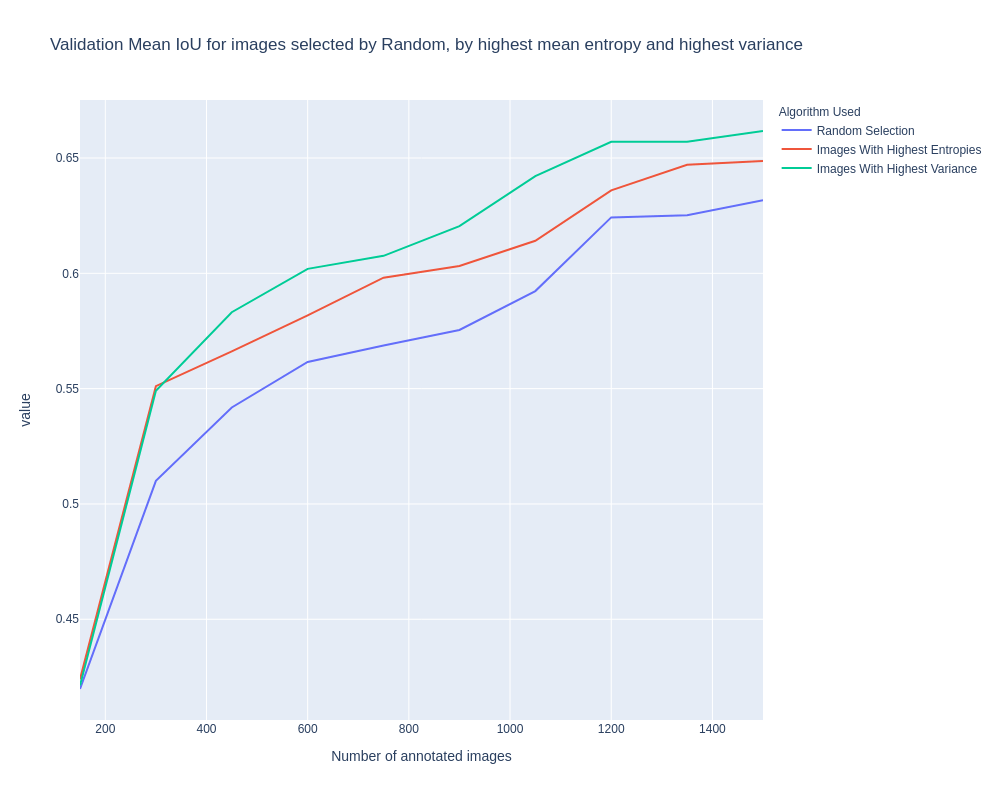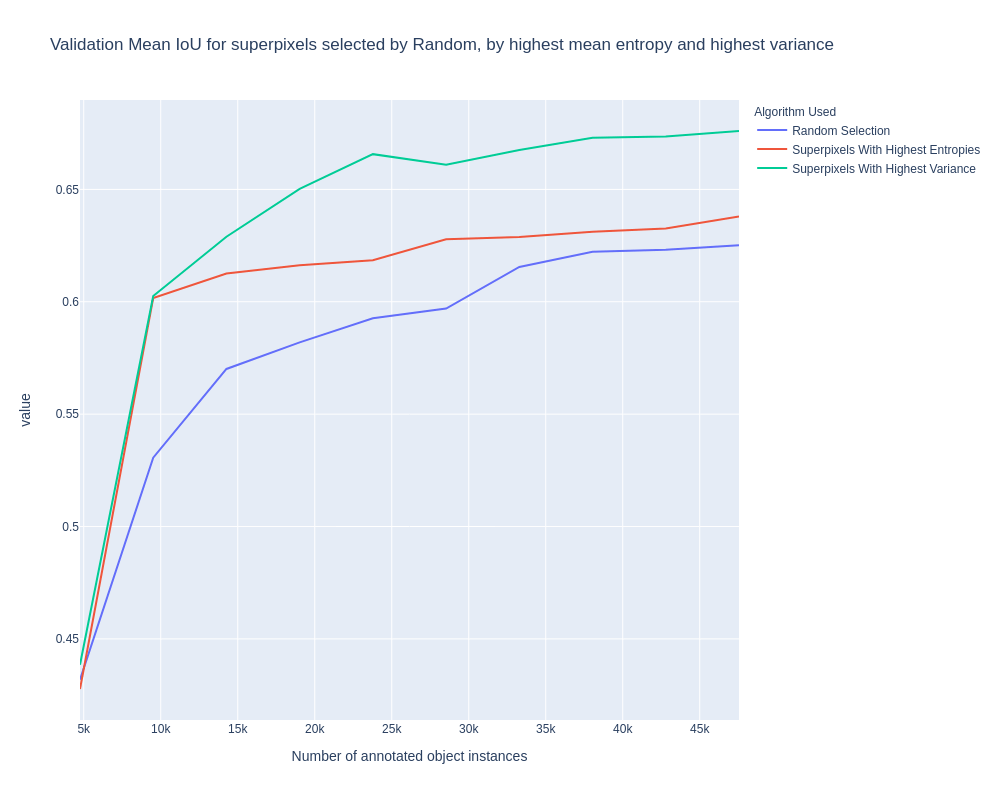Active learning algorithms help deep learning engineers select a subset of images from a large unlabeled pool of data in such a way that obtaining annotations of those images will result in a maximal increase of model accuracy. This is the 3rd article in our list of articles about active learning. In our previous articles, we covered 2 algorithms for using active learning on classification models and results of running loss prediction algorithm on human pose estimation. In the current article we will cover the results of applying different Active Learning methods for semantic segmentation, integration to SuperAnnotate’s platform, share the code and some benchmarking data.

## Used evaluation method

We evaluated the active learning algorithms in 2 scenarios. One scenario assumes selection of images from a pool of unlabeled data, another one divides images into 100 superpixels using the watershed algorithm and selects superpixels to be annotated. In the second scenario the annotator not only provides the class for the selected superpixel, but completely annotates all the object instances which overlap with the given superpixel (most of the time this is going to only 1 object).

We applied several active learning algorithms to select a part of the cityscapes dataset to be annotated and trained the “Dilated Residual Networks” model  on those annotations. Because of the small size of the Cityscapes dataset (2975 images for training), we either select 150 images or 4755 superpixels for 10 cycles (each image on average has 31.7 object instances). The main idea of annotating superpixels is to label only the part of the image, where the given network is “uncertain”, and train it on these partially annotated data. Labeling only a part of the image is much faster than the complete annotation, hence cheaper. If we assume that annotating 1 object instance takes a constant time/money, annotating 4755 objects in many images will take the same effort as fully annotating 150 images.

We used an open-source pytorch implementation  and our modified version can be found here. We run the model “DRN-D-22” which has mean IOU of 0.68 when training on the whole dataset.

## Evaluation of learning loss for active learning algorithm

We tried to apply the “Learning Loss for Active Learning” algorithm for semantic segmentation, even though it’s authors did not report any results for segmentation. We used output features of the 8 levels of the DRN model and the last convolutional and upsampling layers, resulting to features of shapes [ [16, 896, 896], [32, 448, 448], [64, 224, 224], [128, 112, 112], [256, 112, 112], [512, 112, 112], [512, 112, 112], [512, 112, 112], [19, 112, 112], [19, 896, 896]] (CHW format). We saw no improvement in mean IOU when comparing random selection to the loss learning algorithm, yet the ranking accuracy was 0.76 on the validation set, I.E. the algorithm was able to predict the order of the losses fairly well. We also tried to select images using the actual loss values computed using the ground truth labels, and again saw no improvement on the mean IOU. This concludes that selecting 150 images with the highest loss does not result in improved accuracy for segmentation.

## Uncertainty based image selection methods

Another method we evaluated was inspired by the ViewAL algorithm . There are several methods that are based on the prediction of the given model's uncertainty for each pixel, and selecting the images with the highest total uncertainties. The difference of these methods is in the definition of the uncertainty measures.  We start by training our network on 150 randomly selected images, then on each cycle we compute the uncertainty scores for each pixel, sum them up and select 150 images with the highest total uncertainty.

The simplest possible uncertainty measure for the pixel is the entropy of softmax predictions, yet in practice this method did not outperform the random selection. The probable reason for this is the “overconfidence” of the neural networks, resulting in softmax probabilities with high entropies most of the time.

To overcome this  “overconfidence”, Monte Carlo Dropout(MCDR) is being used. Instead of running inference once on each unlabeled image, we run it D= 10 times, while applying an inference time dropout with p=0.5. This way we construct a matrix of size [DxC] for each pixel, where C is the number of classes in the dataset. For cityscapes C=19, because we have 19 classes including the background.

Let’s denote with Pi, d(u,v)(c)our network’s predicted probability for pixel (u,v) of image Ii belonging to class c on run number d. Given this matrix of size [DxC] for each pixel, several uncertainty measures can be developed. One method uses mean values of predictions over the D runs as a probability for a given class, resulting in [C] values per pixel:

Then the entropies of these values are used as an “uncertainty score”:

We tried this method, and the results are provided in Fig. 2. As we can see, the described method is ~1.6% better compared to random when selecting 1500 images, and ~4% better when selecting the first 150 images, after the randomly selected 150.

Another “uncertainty” measure is the mean variance of the D values Pi, d(u,v)(c)for each class C:

You can see a sample uncertainty map for some Cityscapes image in Fig. 1, where darker colors show pixels where the network is more certain. Using these variance instead of the entropy value results in better performance, as shown in Fig. 2. We get validation set mean IoU of 0.6617 when training the network on 1500 images, while training the same network on the whole 2975-image dataset results to mean IoU of 0.68. This outperforms random by ~3%. All the values provided in Fig. 2 and Fig. 4 are the mean of 3 runs with different random seeds. We believe that running the algorithms more than 3 times would result in better evaluation and less randomness in the charts.

While using image-based active learning for segmentation tasks, our customers can create the list of images and their corresponding uncertainties and upload the resulting csv file to the platform.Fig. 2: Results of active learning based on mean Entropy and variance of MC dropout predictions.

## Uncertainty based superpixel selection methods

These methods are similar to the methods described above, except here we divide the image into 100 superpixels with the watershed algorithm, and instead of selecting 150 images we select 4755 superpixels on each cycle. Then annotators will label all the objects that overlap with the given superpixels. The main idea of these methods is to label only parts of the image where the network is uncertain, instead of selecting images for which the mean uncertainty is higher as a result of high uncertainties only in a few parts of it.

Both methods described in the previous section can be easily transformed to select the superpixels, for which the average uncertainty is highest. The same 2 uncertainty measures can be used. Fig. 3 shows our results when using these methods. We can see that both methods outperform random selection on the 1st cycle by a huge 7%, and the variance based method outperforms random selection by ~5% on the last cycle, reaching the mean IoU of 0.676. This means that annotating half of the objects in the images results in almost the same mean IoU value of 0.68, I.E. the annotation effort can be reduced by half with no loss.

When using superpixel based active learning methods we suggest creating pre-annotations for each image with no class labels, and uploading them to our tool as a guide to annotators. Our open-source code provides an example on how to use these methods on the DRN network.Fig. 3: Evaluation results of active learning based on mean Entropy and variance of MC dropout predictions for superpixels selection.

## Concluding remarks

We were able to evaluate several active learning algorithms for segmentation tasks, and the variance of MC dropout methods outperformed the others. We showed that annotation effort can be cut in half with negligible loss to the final model accuracy.

## References

 Donggeun Yoo and In So Kweon. Learning Loss for Active Learning. arXiv:1905.03677 [cs.CV], May 2019.

 Fisher Yu and Vladlen Koltun and Thomas Funkhouser. Dilated Residual Networks. Computer Vision and Pattern Recognition (CVPR) 2017.

 Pytorch implementation of Dilated Residual Networks. https://github.com/fyu/drn

 Yawar Siddiqui, Julien Valentin, Matthias Nießner. ViewAL: Active Learning with Viewpoint Entropy for Semantic Segmentation. arXiv:1911.11789 [cs.CV], Nov 2019.#### Stay connected

Subscribe to receive new blog posts and latest discoveries in the industry from SuperAnnotate